Anúncio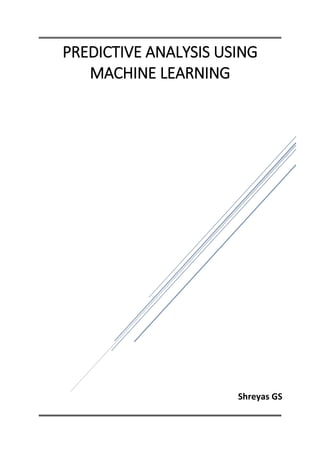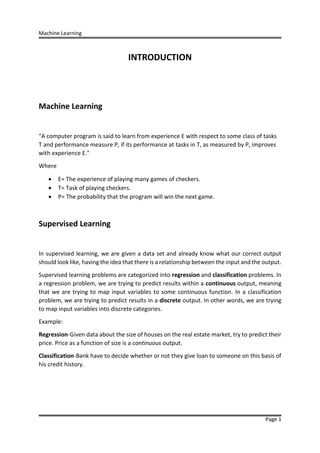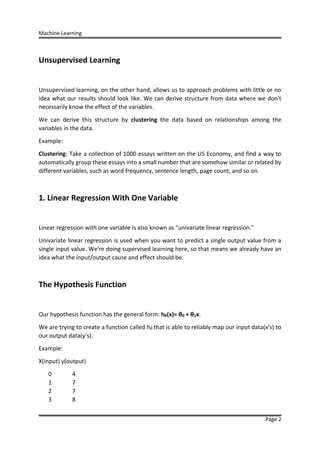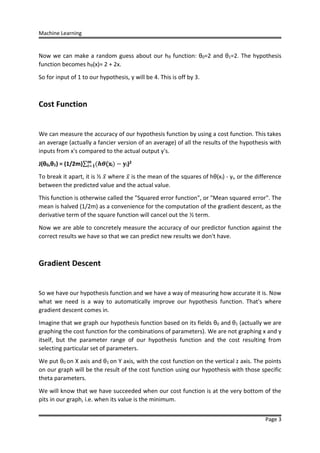Anúncio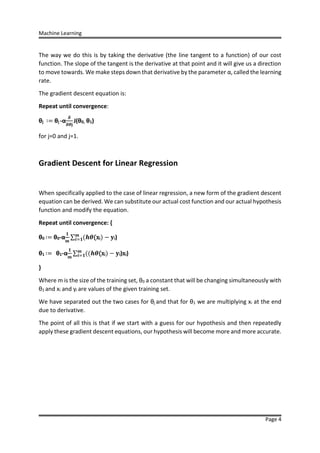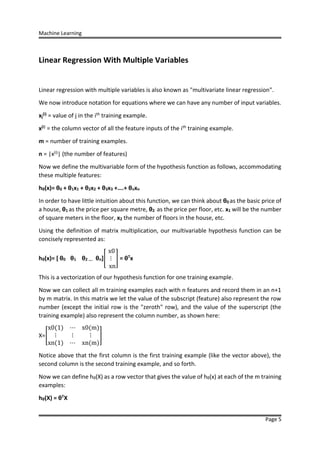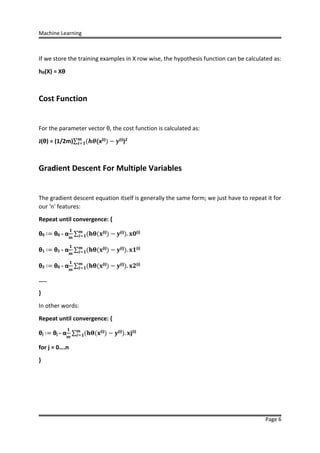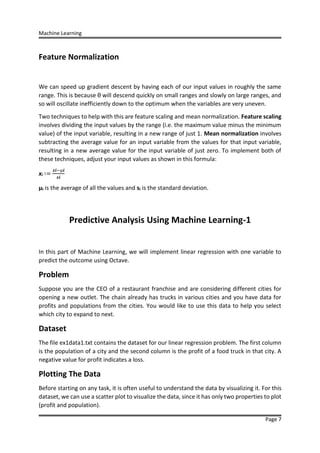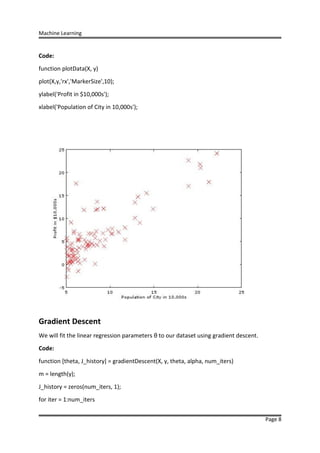Anúncio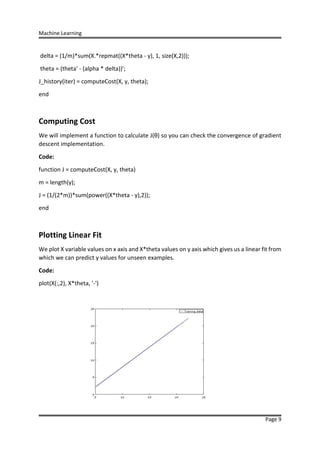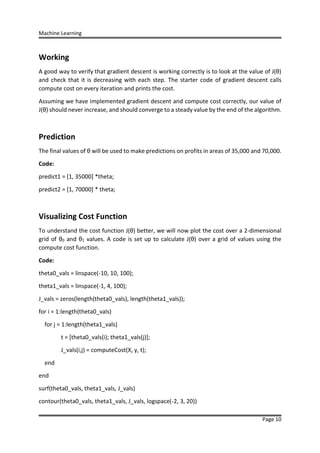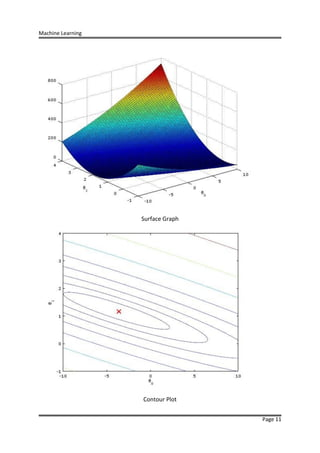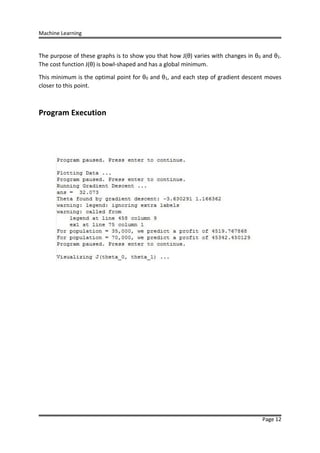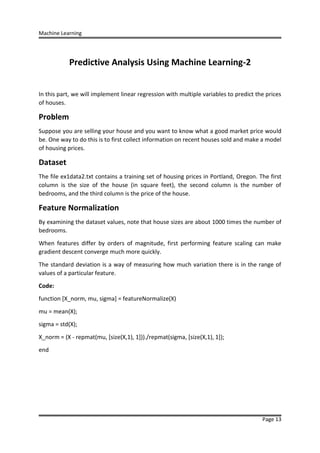Anúncio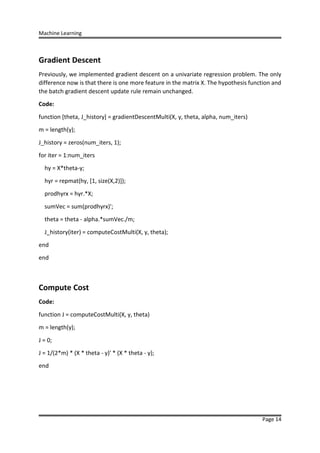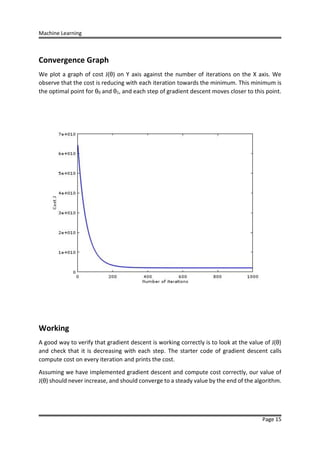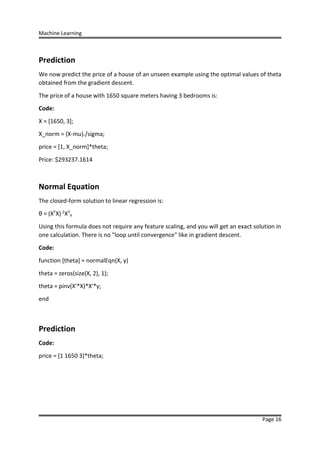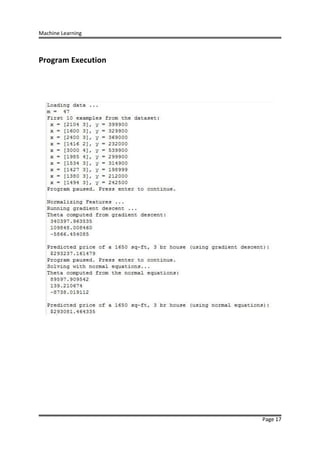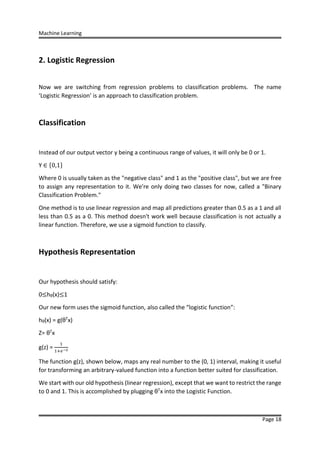Anúncio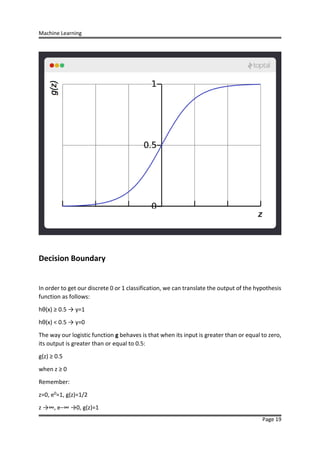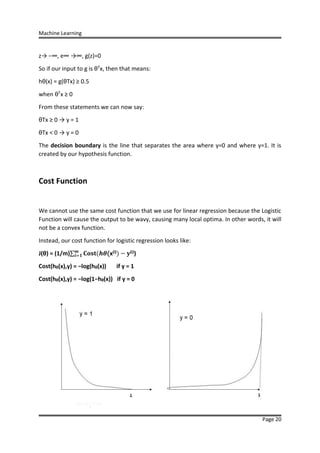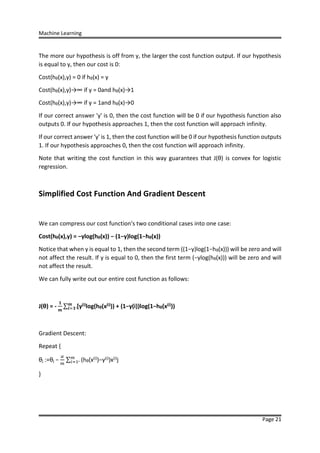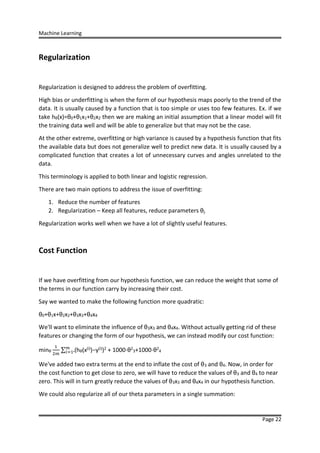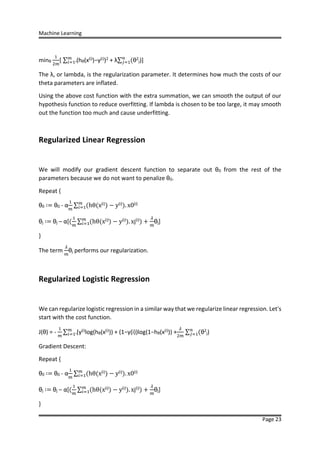Anúncio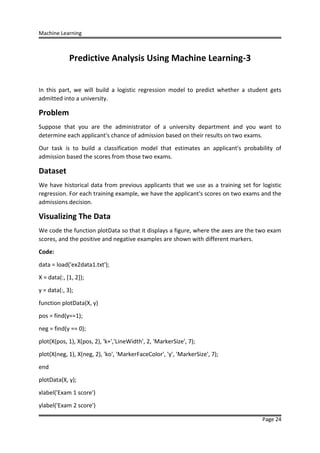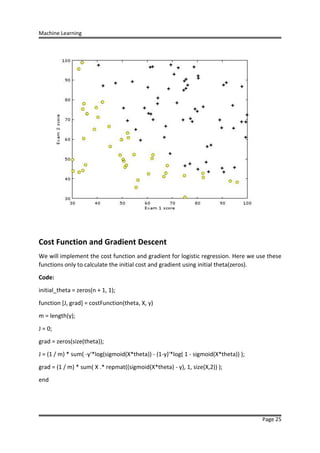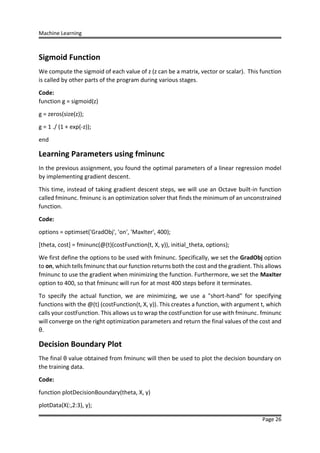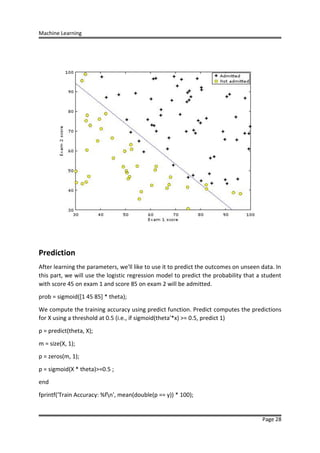Anúncio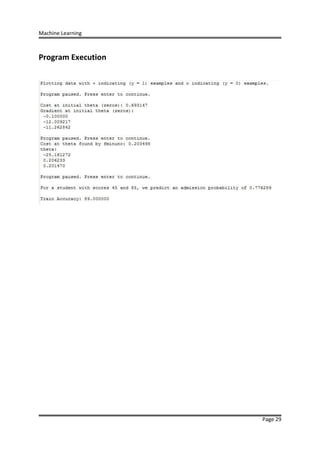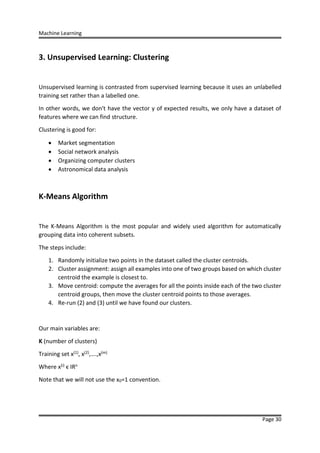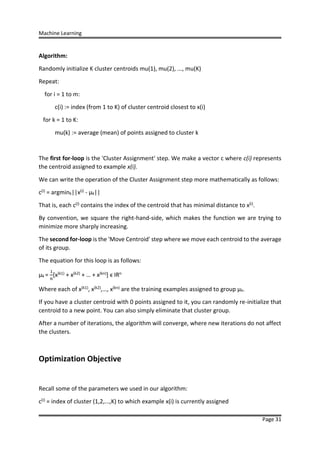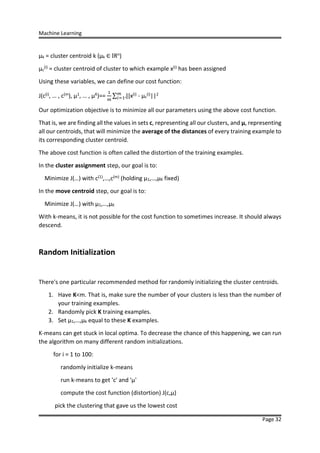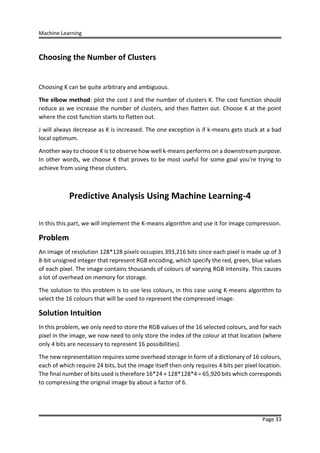Anúncio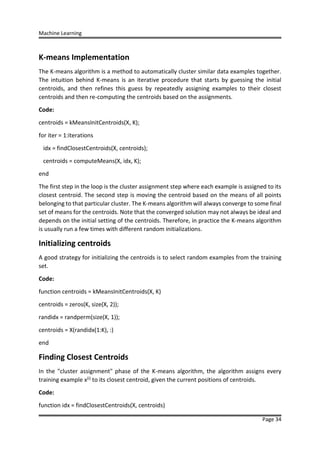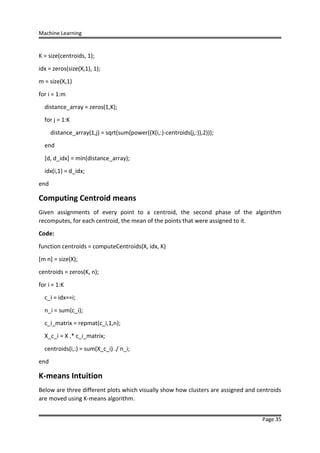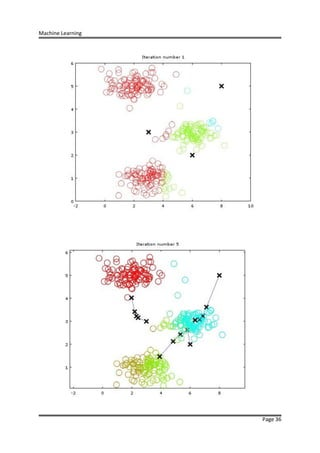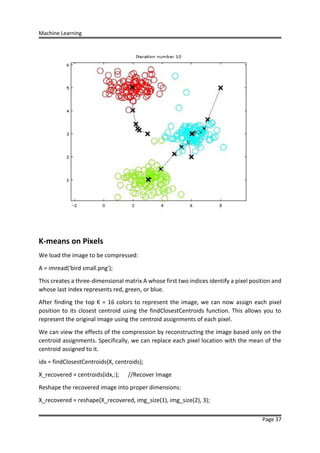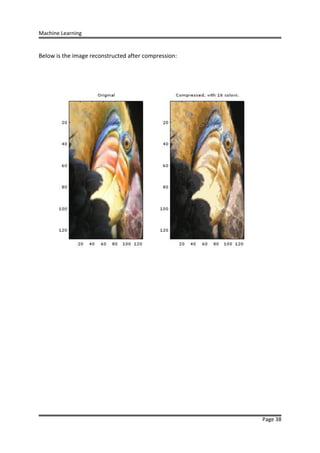Anúncio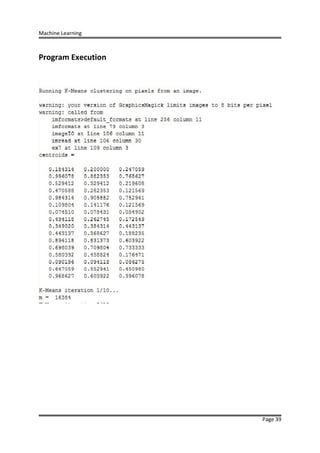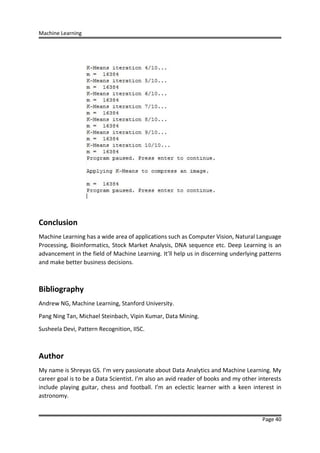Próximos SlideShares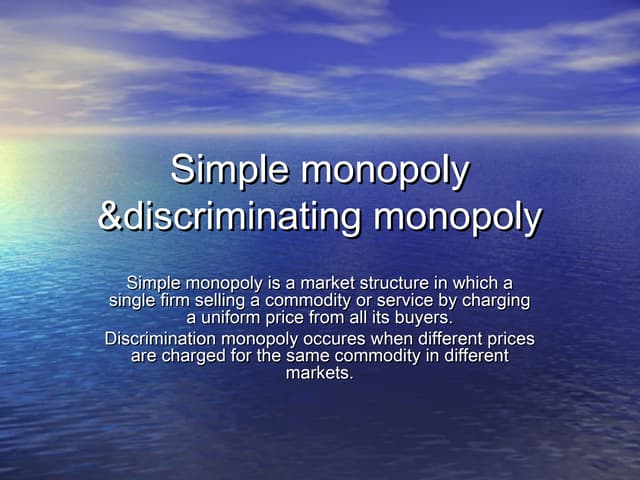Simple monopoly
Carregando em ... 3
1 de 41
Anúncio

### Machine learning

1. Shreyas GS PREDICTIVE ANALYSIS USING MACHINE LEARNING
2. Machine Learning Page 1 INTRODUCTION Machine Learning "A computer program is said to learn from experience E with respect to some class of tasks T and performance measure P, if its performance at tasks in T, as measured by P, improves with experience E." Where  E= The experience of playing many games of checkers.  T= Task of playing checkers.  P= The probability that the program will win the next game. Supervised Learning In supervised learning, we are given a data set and already know what our correct output should look like, having the idea that there is a relationship between the input and the output. Supervised learning problems are categorized into regression and classification problems. In a regression problem, we are trying to predict results within a continuous output, meaning that we are trying to map input variables to some continuous function. In a classification problem, we are trying to predict results in a discrete output. In other words, we are trying to map input variables into discrete categories. Example: Regression-Given data about the size of houses on the real estate market, try to predict their price. Price as a function of size is a continuous output. Classification-Bank have to decide whether or not they give loan to someone on this basis of his credit history.
3. Machine Learning Page 2 Unsupervised Learning Unsupervised learning, on the other hand, allows us to approach problems with little or no idea what our results should look like. We can derive structure from data where we don't necessarily know the effect of the variables. We can derive this structure by clustering the data based on relationships among the variables in the data. Example: Clustering: Take a collection of 1000 essays written on the US Economy, and find a way to automatically group these essays into a small number that are somehow similar or related by different variables, such as word frequency, sentence length, page count, and so on. 1. Linear Regression With One Variable Linear regression with one variable is also known as "univariate linear regression." Univariate linear regression is used when you want to predict a single output value from a single input value. We're doing supervised learning here, so that means we already have an idea what the input/output cause and effect should be. The Hypothesis Function Our hypothesis function has the general form: hθ(x)= θ0 + θ1x. We are trying to create a function called hθ that is able to reliably map our input data(x’s) to our output data(y’s). Example: X(input) y(output) 0 4 1 7 2 7 3 8
4. Machine Learning Page 3 Now we can make a random guess about our hθ function: θ0=2 and θ1=2. The hypothesis function becomes hθ(x)= 2 + 2x. So for input of 1 to our hypothesis, y will be 4. This is off by 3. Cost Function We can measure the accuracy of our hypothesis function by using a cost function. This takes an average (actually a fancier version of an average) of all the results of the hypothesis with inputs from x's compared to the actual output y's. J(θ0,θ1) = (1/2m)∑ (𝒉𝜽(xi 𝒎 𝒊=𝟏 ) − 𝐲i)2 To break it apart, it is ½ 𝑥̅ where 𝑥̅ is the mean of the squares of hθ(xi) - yi, or the difference between the predicted value and the actual value. This function is otherwise called the "Squared error function", or "Mean squared error". The mean is halved (1/2m) as a convenience for the computation of the gradient descent, as the derivative term of the square function will cancel out the ½ term. Now we are able to concretely measure the accuracy of our predictor function against the correct results we have so that we can predict new results we don't have. Gradient Descent So we have our hypothesis function and we have a way of measuring how accurate it is. Now what we need is a way to automatically improve our hypothesis function. That's where gradient descent comes in. Imagine that we graph our hypothesis function based on its fields θ0 and θ1 (actually we are graphing the cost function for the combinations of parameters). We are not graphing x and y itself, but the parameter range of our hypothesis function and the cost resulting from selecting particular set of parameters. We put θ0 on X axis and θ1 on Y axis, with the cost function on the vertical z axis. The points on our graph will be the result of the cost function using our hypothesis with those specific theta parameters. We will know that we have succeeded when our cost function is at the very bottom of the pits in our graph, i.e. when its value is the minimum.
5. Machine Learning Page 4 The way we do this is by taking the derivative (the line tangent to a function) of our cost function. The slope of the tangent is the derivative at that point and it will give us a direction to move towards. We make steps down that derivative by the parameter α, called the learning rate. The gradient descent equation is: Repeat until convergence: θj ∶= θj -α 𝜹 𝜹𝜽𝐣 J(θ0, θ1) for j=0 and j=1. Gradient Descent for Linear Regression When specifically applied to the case of linear regression, a new form of the gradient descent equation can be derived. We can substitute our actual cost function and our actual hypothesis function and modify the equation. Repeat until convergence: { θ0 ∶= θ0-α 𝟏 𝒎 ∑ (𝒉𝜽(xi 𝒎 𝒊=𝟏 ) − 𝐲i) θ1 ∶= θ1-α 𝟏 𝒎 ∑ ((𝒉𝜽(xi 𝒎 𝒊=𝟏 ) − 𝐲i)xi) } Where m is the size of the training set, θ0 a constant that will be changing simultaneously with θ1 and xi and yi are values of the given training set. We have separated out the two cases for θj and that for θ1 we are multiplying xi at the end due to derivative. The point of all this is that if we start with a guess for our hypothesis and then repeatedly apply these gradient descent equations, our hypothesis will become more and more accurate.
6. Machine Learning Page 5 Linear Regression With Multiple Variables Linear regression with multiple variables is also known as "multivariate linear regression". We now introduce notation for equations where we can have any number of input variables. xj (i) = value of j in the ith training example. x(i) = the column vector of all the feature inputs of the ith training example. m = number of training examples. n = |x(i)| (the number of features) Now we define the multivariable form of the hypothesis function as follows, accommodating these multiple features: hθ(x)= θ0 + θ1x1 + θ2x2 + θ3x3 +….+ θnxn In order to have little intuition about this function, we can think about θ0 as the basic price of a house, θ1 as the price per square metre, θ2 as the price per floor, etc. x1 will be the number of square meters in the floor, x2 the number of floors in the house, etc. Using the definition of matrix multiplication, our multivariable hypothesis function can be concisely represented as: hθ(x)= [ θ0 θ1 θ2 …. θn][ x0 ⋮ xn ] = θTx This is a vectorization of our hypothesis function for one training example. Now we can collect all m training examples each with n features and record them in an n+1 by m matrix. In this matrix we let the value of the subscript (feature) also represent the row number (except the initial row is the "zeroth" row), and the value of the superscript (the training example) also represent the column number, as shown here: X=[ x0(1) ⋯ x0(m) ⋮ ⋮ ⋮ xn(1) ⋯ xn(m) ] Notice above that the first column is the first training example (like the vector above), the second column is the second training example, and so forth. Now we can define hθ(X) as a row vector that gives the value of hθ(x) at each of the m training examples: hθ(X) = θTX
7. Machine Learning Page 6 If we store the training examples in X row wise, the hypothesis function can be calculated as: hθ(X) = Xθ Cost Function For the parameter vector θ, the cost function is calculated as: J(θ) = (1/2m)∑ (𝒉𝜽(x(i)𝒎 𝒊=𝟏 ) − 𝐲(i))2 Gradient Descent For Multiple Variables The gradient descent equation itself is generally the same form; we just have to repeat it for our 'n' features: Repeat until convergence: { θ0 ∶= θ0 - α 𝟏 𝒎 ∑ (𝐡𝛉(𝐱(i)) − 𝐲(i)𝒎 𝒊=𝟏 ). 𝐱𝟎(i) θ1 ∶= θ1 - α 𝟏 𝒎 ∑ (𝐡𝛉(𝐱(i)) − 𝐲(i)𝒎 𝒊=𝟏 ). 𝐱𝟏(i) θ2 ∶= θ0 - α 𝟏 𝒎 ∑ (𝐡𝛉(𝐱(i)) − 𝐲(i)𝒎 𝒊=𝟏 ). 𝐱𝟐(i) ….. } In other words: Repeat until convergence: { θj ∶= θj - α 𝟏 𝒎 ∑ (𝐡𝛉(𝐱(i)) − 𝐲(i)𝒎 𝒊=𝟏 ). 𝐱𝐣(i) for j = 0….n }
8. Machine Learning Page 7 Feature Normalization We can speed up gradient descent by having each of our input values in roughly the same range. This is because θ will descend quickly on small ranges and slowly on large ranges, and so will oscillate inefficiently down to the optimum when the variables are very uneven. Two techniques to help with this are feature scaling and mean normalization. Feature scaling involves dividing the input values by the range (i.e. the maximum value minus the minimum value) of the input variable, resulting in a new range of just 1. Mean normalization involves subtracting the average value for an input variable from the values for that input variable, resulting in a new average value for the input variable of just zero. To implement both of these techniques, adjust your input values as shown in this formula: xi ∶= 𝒙𝒊−µ𝒊 𝒔𝒊 µi is the average of all the values and si is the standard deviation. Predictive Analysis Using Machine Learning-1 In this part of Machine Learning, we will implement linear regression with one variable to predict the outcome using Octave. Problem Suppose you are the CEO of a restaurant franchise and are considering different cities for opening a new outlet. The chain already has trucks in various cities and you have data for profits and populations from the cities. You would like to use this data to help you select which city to expand to next. Dataset The file ex1data1.txt contains the dataset for our linear regression problem. The first column is the population of a city and the second column is the profit of a food truck in that city. A negative value for profit indicates a loss. Plotting The Data Before starting on any task, it is often useful to understand the data by visualizing it. For this dataset, we can use a scatter plot to visualize the data, since it has only two properties to plot (profit and population).
9. Machine Learning Page 8 Code: function plotData(X, y) plot(X,y,'rx','MarkerSize',10); ylabel('Profit in $10,000s'); xlabel('Population of City in 10,000s'); Gradient Descent We will fit the linear regression parameters θ to our dataset using gradient descent. Code: function [theta, J_history] = gradientDescent(X, y, theta, alpha, num_iters) m = length(y); J_history = zeros(num_iters, 1); for iter = 1:num_iters 10. Machine Learning Page 9 delta = (1/m)*sum(X.*repmat((X*theta - y), 1, size(X,2))); theta = (theta' - (alpha * delta))'; J_history(iter) = computeCost(X, y, theta); end Computing Cost We will implement a function to calculate J(θ) so you can check the convergence of gradient descent implementation. Code: function J = computeCost(X, y, theta) m = length(y); J = (1/(2*m))*sum(power((X*theta - y),2)); end Plotting Linear Fit We plot X variable values on x axis and X*theta values on y axis which gives us a linear fit from which we can predict y values for unseen examples. Code: plot(X(:,2), X*theta, '-') 11. Machine Learning Page 10 Working A good way to verify that gradient descent is working correctly is to look at the value of J(θ) and check that it is decreasing with each step. The starter code of gradient descent calls compute cost on every iteration and prints the cost. Assuming we have implemented gradient descent and compute cost correctly, our value of J(θ) should never increase, and should converge to a steady value by the end of the algorithm. Prediction The final values of θ will be used to make predictions on profits in areas of 35,000 and 70,000. Code: predict1 = [1, 35000] *theta; predict2 = [1, 70000] * theta; Visualizing Cost Function To understand the cost function J(θ) better, we will now plot the cost over a 2-dimensional grid of θ0 and θ1 values. A code is set up to calculate J(θ) over a grid of values using the compute cost function. Code: theta0_vals = linspace(-10, 10, 100); theta1_vals = linspace(-1, 4, 100); J_vals = zeros(length(theta0_vals), length(theta1_vals)); for i = 1:length(theta0_vals) for j = 1:length(theta1_vals) t = [theta0_vals(i); theta1_vals(j)]; J_vals(i,j) = computeCost(X, y, t); end end surf(theta0_vals, theta1_vals, J_vals) contour(theta0_vals, theta1_vals, J_vals, logspace(-2, 3, 20)) 12. Machine Learning Page 11 Surface Graph Contour Plot 13. Machine Learning Page 12 The purpose of these graphs is to show you that how J(θ) varies with changes in θ0 and θ1. The cost function J(θ) is bowl-shaped and has a global minimum. This minimum is the optimal point for θ0 and θ1, and each step of gradient descent moves closer to this point. Program Execution 14. Machine Learning Page 13 Predictive Analysis Using Machine Learning-2 In this part, we will implement linear regression with multiple variables to predict the prices of houses. Problem Suppose you are selling your house and you want to know what a good market price would be. One way to do this is to first collect information on recent houses sold and make a model of housing prices. Dataset The file ex1data2.txt contains a training set of housing prices in Portland, Oregon. The first column is the size of the house (in square feet), the second column is the number of bedrooms, and the third column is the price of the house. Feature Normalization By examining the dataset values, note that house sizes are about 1000 times the number of bedrooms. When features differ by orders of magnitude, first performing feature scaling can make gradient descent converge much more quickly. The standard deviation is a way of measuring how much variation there is in the range of values of a particular feature. Code: function [X_norm, mu, sigma] = featureNormalize(X) mu = mean(X); sigma = std(X); X_norm = (X - repmat(mu, [size(X,1), 1]))./repmat(sigma, [size(X,1), 1]); end 15. Machine Learning Page 14 Gradient Descent Previously, we implemented gradient descent on a univariate regression problem. The only difference now is that there is one more feature in the matrix X. The hypothesis function and the batch gradient descent update rule remain unchanged. Code: function [theta, J_history] = gradientDescentMulti(X, y, theta, alpha, num_iters) m = length(y); J_history = zeros(num_iters, 1); for iter = 1:num_iters hy = X*theta-y; hyr = repmat(hy, [1, size(X,2)]); prodhyrx = hyr.*X; sumVec = sum(prodhyrx)'; theta = theta - alpha.*sumVec./m; J_history(iter) = computeCostMulti(X, y, theta); end end Compute Cost Code: function J = computeCostMulti(X, y, theta) m = length(y); J = 0; J = 1/(2*m) * (X * theta - y)' * (X * theta - y); end 16. Machine Learning Page 15 Convergence Graph We plot a graph of cost J(θ) on Y axis against the number of iterations on the X axis. We observe that the cost is reducing with each iteration towards the minimum. This minimum is the optimal point for θ0 and θ1, and each step of gradient descent moves closer to this point. Working A good way to verify that gradient descent is working correctly is to look at the value of J(θ) and check that it is decreasing with each step. The starter code of gradient descent calls compute cost on every iteration and prints the cost. Assuming we have implemented gradient descent and compute cost correctly, our value of J(θ) should never increase, and should converge to a steady value by the end of the algorithm. 17. Machine Learning Page 16 Prediction We now predict the price of a house of an unseen example using the optimal values of theta obtained from the gradient descent. The price of a house with 1650 square meters having 3 bedrooms is: Code: X = [1650, 3]; X_norm = (X-mu)./sigma; price = [1, X_norm]*theta; Price:$293237.1614 Normal Equation The closed-form solution to linear regression is: θ = (XTX)-1XT y Using this formula does not require any feature scaling, and you will get an exact solution in one calculation. There is no "loop until convergence" like in gradient descent. Code: function [theta] = normalEqn(X, y) theta = zeros(size(X, 2), 1); theta = pinv(X'*X)*X'*y; end Prediction Code: price = [1 1650 3]*theta;
18. Machine Learning Page 17 Program Execution
19. Machine Learning Page 18 2. Logistic Regression Now we are switching from regression problems to classification problems. The name ‘Logistic Regression’ is an approach to classification problem. Classification Instead of our output vector y being a continuous range of values, it will only be 0 or 1. Y ∈ {0,1} Where 0 is usually taken as the "negative class" and 1 as the "positive class", but we are free to assign any representation to it. We're only doing two classes for now, called a "Binary Classification Problem." One method is to use linear regression and map all predictions greater than 0.5 as a 1 and all less than 0.5 as a 0. This method doesn't work well because classification is not actually a linear function. Therefore, we use a sigmoid function to classify. Hypothesis Representation Our hypothesis should satisfy: 0≤hθ(x)≤1 Our new form uses the sigmoid function, also called the “logistic function”: hθ(x) = g(θTx) Z= θTx g(z) = 1 1+𝑒−𝑧 The function g(z), shown below, maps any real number to the (0, 1) interval, making it useful for transforming an arbitrary-valued function into a function better suited for classification. We start with our old hypothesis (linear regression), except that we want to restrict the range to 0 and 1. This is accomplished by plugging θTx into the Logistic Function.
20. Machine Learning Page 19 Decision Boundary In order to get our discrete 0 or 1 classification, we can translate the output of the hypothesis function as follows: hθ(x) ≥ 0.5 → y=1 hθ(x) < 0.5 → y=0 The way our logistic function g behaves is that when its input is greater than or equal to zero, its output is greater than or equal to 0.5: g(z) ≥ 0.5 when z ≥ 0 Remember: z=0, e0=1, g(z)=1/2 z →∞, e−∞ →0, g(z)=1
21. Machine Learning Page 20 z→ −∞, e∞ →∞, g(z)=0 So if our input to g is θTx, then that means: hθ(x) = g(θTx) ≥ 0.5 when θTx ≥ 0 From these statements we can now say: θTx ≥ 0 → y = 1 θTx < 0 → y = 0 The decision boundary is the line that separates the area where y=0 and where y=1. It is created by our hypothesis function. Cost Function We cannot use the same cost function that we use for linear regression because the Logistic Function will cause the output to be wavy, causing many local optima. In other words, it will not be a convex function. Instead, our cost function for logistic regression looks like: J(θ) = (1/m)∑ 𝐂𝐨𝐬𝐭(𝒉𝜽(x(i)𝒎 𝒊=𝟏 ) − 𝐲(i)) Cost(hθ(x),y) = −log(hθ(x)) if y = 1 Cost(hθ(x),y) = −log(1−hθ(x)) if y = 0
22. Machine Learning Page 21 The more our hypothesis is off from y, the larger the cost function output. If our hypothesis is equal to y, then our cost is 0: Cost(hθ(x),y) = 0 if hθ(x) = y Cost(hθ(x),y)→∞ if y = 0and hθ(x)→1 Cost(hθ(x),y)→∞ if y = 1and hθ(x)→0 If our correct answer 'y' is 0, then the cost function will be 0 if our hypothesis function also outputs 0. If our hypothesis approaches 1, then the cost function will approach infinity. If our correct answer 'y' is 1, then the cost function will be 0 if our hypothesis function outputs 1. If our hypothesis approaches 0, then the cost function will approach infinity. Note that writing the cost function in this way guarantees that J(θ) is convex for logistic regression. Simplified Cost Function And Gradient Descent We can compress our cost function's two conditional cases into one case: Cost(hθ(x),y) = −ylog(hθ(x)) − (1−y)log(1−hθ(x)) Notice that when y is equal to 1, then the second term ((1−y)log(1−hθ(x))) will be zero and will not affect the result. If y is equal to 0, then the first term (−ylog(hθ(x))) will be zero and will not affect the result. We can fully write out our entire cost function as follows: J(θ) = - 𝟏 𝒎 ∑ .𝒎 𝒊=𝟏 [y(i)log(hθ(x(i))) + (1−y(i))log(1−hθ(x(i))) Gradient Descent: Repeat { θj :=θj − 𝛼 𝑚 ∑ .𝑚 𝑖=1 (hθ(x(i))−y(i))x(i)j }
23. Machine Learning Page 22 Regularization Regularization is designed to address the problem of overfitting. High bias or underfitting is when the form of our hypothesis maps poorly to the trend of the data. It is usually caused by a function that is too simple or uses too few features. Ex. if we take hθ(x)=θ0+θ1x1+θ2x2 then we are making an initial assumption that a linear model will fit the training data well and will be able to generalize but that may not be the case. At the other extreme, overfitting or high variance is caused by a hypothesis function that fits the available data but does not generalize well to predict new data. It is usually caused by a complicated function that creates a lot of unnecessary curves and angles unrelated to the data. This terminology is applied to both linear and logistic regression. There are two main options to address the issue of overfitting: 1. Reduce the number of features 2. Regularization – Keep all features, reduce parameters θj. Regularization works well when we have a lot of slightly useful features. Cost Function If we have overfitting from our hypothesis function, we can reduce the weight that some of the terms in our function carry by increasing their cost. Say we wanted to make the following function more quadratic: θ0+θ1x+θ2x2+θ3x3+θ4x4 We'll want to eliminate the influence of θ3x3 and θ4x4. Without actually getting rid of these features or changing the form of our hypothesis, we can instead modify our cost function: minθ 1 2𝑚 ∑ .𝑚 𝑖=1 (hθ(x(i))−y(i))2 + 1000⋅θ2 3+1000⋅θ2 4 We've added two extra terms at the end to inflate the cost of θ3 and θ4. Now, in order for the cost function to get close to zero, we will have to reduce the values of θ3 and θ4 to near zero. This will in turn greatly reduce the values of θ3x3 and θ4x4 in our hypothesis function. We could also regularize all of our theta parameters in a single summation:
24. Machine Learning Page 23 minθ 1 2𝑚 [ ∑ .𝑚 𝑖=1 (hθ(x(i))−y(i))2 + λ∑ (𝑛 𝑗=1 θ2 j)] The λ, or lambda, is the regularization parameter. It determines how much the costs of our theta parameters are inflated. Using the above cost function with the extra summation, we can smooth the output of our hypothesis function to reduce overfitting. If lambda is chosen to be too large, it may smooth out the function too much and cause underfitting. Regularized Linear Regression We will modify our gradient descent function to separate out θ0 from the rest of the parameters because we do not want to penalize θ0. Repeat { θ0 ∶= θ0 - α 1 𝑚 ∑ (hθ(x(i)) − y(i)𝑚 𝑖=1 ). x0(i) θj ∶= θj – α[( 1 𝑚 ∑ (hθ(x(i)) − y(i)𝑚 𝑖=1 ). xj(i)) + 𝜆 𝑚 θj] } The term 𝜆 𝑚 θj performs our regularization. Regularized Logistic Regression We can regularize logistic regression in a similar way that we regularize linear regression. Let's start with the cost function. J(θ) = - 1 𝑚 ∑ .𝑚 𝑖=1 [y(i)log(hθ(x(i))) + (1−y(i))log(1−hθ(x(i))) + 𝜆 2𝑚 ∑ (𝑛 𝑗=1 θ2 j) Gradient Descent: Repeat { θ0 ∶= θ0 - α 1 𝑚 ∑ (hθ(x(i)) − y(i)𝑚 𝑖=1 ). x0(i) θj ∶= θj – α[( 1 𝑚 ∑ (hθ(x(i)) − y(i)𝑚 𝑖=1 ). xj(i)) + 𝜆 𝑚 θj] }
25. Machine Learning Page 24 Predictive Analysis Using Machine Learning-3 In this part, we will build a logistic regression model to predict whether a student gets admitted into a university. Problem Suppose that you are the administrator of a university department and you want to determine each applicant's chance of admission based on their results on two exams. Our task is to build a classification model that estimates an applicant's probability of admission based the scores from those two exams. Dataset We have historical data from previous applicants that we use as a training set for logistic regression. For each training example, we have the applicant's scores on two exams and the admissions decision. Visualizing The Data We code the function plotData so that it displays a figure, where the axes are the two exam scores, and the positive and negative examples are shown with different markers. Code: data = load('ex2data1.txt'); X = data(:, [1, 2]); y = data(:, 3); function plotData(X, y) pos = find(y==1); neg = find(y == 0); plot(X(pos, 1), X(pos, 2), 'k+','LineWidth', 2, 'MarkerSize', 7); plot(X(neg, 1), X(neg, 2), 'ko', 'MarkerFaceColor', 'y', 'MarkerSize', 7); end plotData(X, y); xlabel('Exam 1 score') ylabel('Exam 2 score')
26. Machine Learning Page 25 Cost Function and Gradient Descent We will implement the cost function and gradient for logistic regression. Here we use these functions only to calculate the initial cost and gradient using initial theta(zeros). Code: initial_theta = zeros(n + 1, 1); function [J, grad] = costFunction(theta, X, y) m = length(y); J = 0; grad = zeros(size(theta)); J = (1 / m) * sum( -y'*log(sigmoid(X*theta)) - (1-y)'*log( 1 - sigmoid(X*theta)) ); grad = (1 / m) * sum( X .* repmat((sigmoid(X*theta) - y), 1, size(X,2)) ); end
27. Machine Learning Page 26 Sigmoid Function We compute the sigmoid of each value of z (z can be a matrix, vector or scalar). This function is called by other parts of the program during various stages. Code: function g = sigmoid(z) g = zeros(size(z)); g = 1 ./ (1 + exp(-z)); end Learning Parameters using fminunc In the previous assignment, you found the optimal parameters of a linear regression model by implementing gradient descent. This time, instead of taking gradient descent steps, we will use an Octave built-in function called fminunc. fminunc is an optimization solver that finds the minimum of an unconstrained function. Code: options = optimset('GradObj', 'on', 'MaxIter', 400); [theta, cost] = fminunc(@(t)(costFunction(t, X, y)), initial_theta, options); We first define the options to be used with fminunc. Specifically, we set the GradObj option to on, which tells fminunc that our function returns both the cost and the gradient. This allows fminunc to use the gradient when minimizing the function. Furthermore, we set the MaxIter option to 400, so that fminunc will run for at most 400 steps before it terminates. To specify the actual function, we are minimizing, we use a "short-hand" for specifying functions with the @(t) (costFunction(t, X, y)). This creates a function, with argument t, which calls your costFunction. This allows us to wrap the costFunction for use with fminunc. fminunc will converge on the right optimization parameters and return the final values of the cost and θ. Decision Boundary Plot The final θ value obtained from fminunc will then be used to plot the decision boundary on the training data. Code: function plotDecisionBoundary(theta, X, y) plotData(X(:,2:3), y);
28. Machine Learning Page 27 hold on if size(X, 2) <= 3 plot_x = [min(X(:,2))-2, max(X(:,2))+2]; plot_y = (-1./theta(3)).*(theta(2).*plot_x + theta(1)); plot(plot_x, plot_y) legend('Admitted', 'Not admitted', 'Decision Boundary') axis([30, 100, 30, 100]) else % Here is the grid range u = linspace(-1, 1.5, 50); v = linspace(-1, 1.5, 50); z = zeros(length(u), length(v)); for i = 1:length(u) for j = 1:length(v) z(i,j) = mapFeature(u(i), v(j))*theta; end end z = z'; contour(u, v, z, [0, 0], 'LineWidth', 2) end hold off end plotDecisionBoundary plots the data points X and y into a new figure with the decision boundary defined by theta.
29. Machine Learning Page 28 Prediction After learning the parameters, we'll like to use it to predict the outcomes on unseen data. In this part, we will use the logistic regression model to predict the probability that a student with score 45 on exam 1 and score 85 on exam 2 will be admitted. prob = sigmoid([1 45 85] * theta); We compute the training accuracy using predict function. Predict computes the predictions for X using a threshold at 0.5 (i.e., if sigmoid(theta'*x) >= 0.5, predict 1) p = predict(theta, X); m = size(X, 1); p = zeros(m, 1); p = sigmoid(X * theta)>=0.5 ; end fprintf('Train Accuracy: %fn', mean(double(p == y)) * 100);
30. Machine Learning Page 29 Program Execution
31. Machine Learning Page 30 3. Unsupervised Learning: Clustering Unsupervised learning is contrasted from supervised learning because it uses an unlabelled training set rather than a labelled one. In other words, we don't have the vector y of expected results, we only have a dataset of features where we can find structure. Clustering is good for:  Market segmentation  Social network analysis  Organizing computer clusters  Astronomical data analysis K-Means Algorithm The K-Means Algorithm is the most popular and widely used algorithm for automatically grouping data into coherent subsets. The steps include: 1. Randomly initialize two points in the dataset called the cluster centroids. 2. Cluster assignment: assign all examples into one of two groups based on which cluster centroid the example is closest to. 3. Move centroid: compute the averages for all the points inside each of the two cluster centroid groups, then move the cluster centroid points to those averages. 4. Re-run (2) and (3) until we have found our clusters. Our main variables are: K (number of clusters) Training set x(1), x(2),….,x(m) Where x(i) ϵ IRn Note that we will not use the x0=1 convention.
32. Machine Learning Page 31 Algorithm: Randomly initialize K cluster centroids mu(1), mu(2), ..., mu(K) Repeat: for i = 1 to m: c(i) := index (from 1 to K) of cluster centroid closest to x(i) for k = 1 to K: mu(k) := average (mean) of points assigned to cluster k The first for-loop is the 'Cluster Assignment' step. We make a vector c where c(i) represents the centroid assigned to example x(i). We can write the operation of the Cluster Assignment step more mathematically as follows: c(i) = argmink||x(i) - µk|| That is, each c(i) contains the index of the centroid that has minimal distance to x(i). By convention, we square the right-hand-side, which makes the function we are trying to minimize more sharply increasing. The second for-loop is the 'Move Centroid' step where we move each centroid to the average of its group. The equation for this loop is as follows: µk = 1 𝑛 [x(k1) + x(k2) + … + x(kn)] ϵ IRn Where each of x(k1), x(k2),…, x(kn) are the training examples assigned to group μk. If you have a cluster centroid with 0 points assigned to it, you can randomly re-initialize that centroid to a new point. You can also simply eliminate that cluster group. After a number of iterations, the algorithm will converge, where new iterations do not affect the clusters. Optimization Objective Recall some of the parameters we used in our algorithm: c(i) = index of cluster (1,2,...,K) to which example x(i) is currently assigned
33. Machine Learning Page 32 μk = cluster centroid k (μk ∈ IRn) μc (i) = cluster centroid of cluster to which example x(i) has been assigned Using these variables, we can define our cost function: J(c(i), … , c(m), μ1, … , μK)== 1 𝑚 ∑ .𝑚 𝑖=1 ||x(i) - μc (i)||2 Our optimization objective is to minimize all our parameters using the above cost function. That is, we are finding all the values in sets c, representing all our clusters, and μ, representing all our centroids, that will minimize the average of the distances of every training example to its corresponding cluster centroid. The above cost function is often called the distortion of the training examples. In the cluster assignment step, our goal is to: Minimize J(…) with c(1),…,c(m) (holding μ1,…,μK fixed) In the move centroid step, our goal is to: Minimize J(…) with μ1,…,μK With k-means, it is not possible for the cost function to sometimes increase. It should always descend. Random Initialization There's one particular recommended method for randomly initializing the cluster centroids. 1. Have K<m. That is, make sure the number of your clusters is less than the number of your training examples. 2. Randomly pick K training examples. 3. Set μ1,…,μk equal to these K examples. K-means can get stuck in local optima. To decrease the chance of this happening, we can run the algorithm on many different random initializations. for i = 1 to 100: randomly initialize k-means run k-means to get 'c' and 'µ' compute the cost function (distortion) J(c,µ) pick the clustering that gave us the lowest cost
34. Machine Learning Page 33 Choosing the Number of Clusters Choosing K can be quite arbitrary and ambiguous. The elbow method: plot the cost J and the number of clusters K. The cost function should reduce as we increase the number of clusters, and then flatten out. Choose K at the point where the cost function starts to flatten out. J will always decrease as K is increased. The one exception is if k-means gets stuck at a bad local optimum. Another way to choose K is to observe how well k-means performs on a downstream purpose. In other words, we choose K that proves to be most useful for some goal you're trying to achieve from using these clusters. Predictive Analysis Using Machine Learning-4 In this this part, we will implement the K-means algorithm and use it for image compression. Problem An image of resolution 128*128 pixels occupies 393,216 bits since each pixel is made up of 3 8-bit unsigned integer that represent RGB encoding, which specify the red, green, blue values of each pixel. The image contains thousands of colours of varying RGB intensity. This causes a lot of overhead on memory for storage. The solution to this problem is to use less colours, in this case using K-means algorithm to select the 16 colours that will be used to represent the compressed image. Solution Intuition In this problem, we only need to store the RGB values of the 16 selected colours, and for each pixel in the image, we now need to only store the index of the colour at that location (where only 4 bits are necessary to represent 16 possibilities). The new representation requires some overhead storage in form of a dictionary of 16 colours, each of which require 24 bits, but the image itself then only requires 4 bits per pixel location. The final number of bits used is therefore 16*24 + 128*128*4 = 65,920 bits which corresponds to compressing the original image by about a factor of 6.
35. Machine Learning Page 34 K-means Implementation The K-means algorithm is a method to automatically cluster similar data examples together. The intuition behind K-means is an iterative procedure that starts by guessing the initial centroids, and then refines this guess by repeatedly assigning examples to their closest centroids and then re-computing the centroids based on the assignments. Code: centroids = kMeansInitCentroids(X, K); for iter = 1:iterations idx = findClosestCentroids(X, centroids); centroids = computeMeans(X, idx, K); end The first step in the loop is the cluster assignment step where each example is assigned to its closest centroid. The second step is moving the centroid based on the means of all points belonging to that particular cluster. The K-means algorithm will always converge to some final set of means for the centroids. Note that the converged solution may not always be ideal and depends on the initial setting of the centroids. Therefore, in practice the K-means algorithm is usually run a few times with different random initializations. Initializing centroids A good strategy for initializing the centroids is to select random examples from the training set. Code: function centroids = kMeansInitCentroids(X, K) centroids = zeros(K, size(X, 2)); randidx = randperm(size(X, 1)); centroids = X(randidx(1:K), :) end Finding Closest Centroids In the "cluster assignment" phase of the K-means algorithm, the algorithm assigns every training example x(i) to its closest centroid, given the current positions of centroids. Code: function idx = findClosestCentroids(X, centroids)
36. Machine Learning Page 35 K = size(centroids, 1); idx = zeros(size(X,1), 1); m = size(X,1) for i = 1:m distance_array = zeros(1,K); for j = 1:K distance_array(1,j) = sqrt(sum(power((X(i,:)-centroids(j,:)),2))); end [d, d_idx] = min(distance_array); idx(i,1) = d_idx; end Computing Centroid means Given assignments of every point to a centroid, the second phase of the algorithm recomputes, for each centroid, the mean of the points that were assigned to it. Code: function centroids = computeCentroids(X, idx, K) [m n] = size(X); centroids = zeros(K, n); for i = 1:K c_i = idx==i; n_i = sum(c_i); c_i_matrix = repmat(c_i,1,n); X_c_i = X .* c_i_matrix; centroids(i,:) = sum(X_c_i) ./ n_i; end K-means Intuition Below are three different plots which visually show how clusters are assigned and centroids are moved using K-means algorithm.
37. Machine Learning Page 36
38. Machine Learning Page 37 K-means on Pixels We load the image to be compressed: A = imread('bird small.png'); This creates a three-dimensional matrix A whose first two indices identify a pixel position and whose last index represents red, green, or blue. After finding the top K = 16 colors to represent the image, we can now assign each pixel position to its closest centroid using the findClosestCentroids function. This allows you to represent the original image using the centroid assignments of each pixel. We can view the effects of the compression by reconstructing the image based only on the centroid assignments. Specifically, we can replace each pixel location with the mean of the centroid assigned to it. idx = findClosestCentroids(X, centroids); X_recovered = centroids(idx,:); //Recover Image Reshape the recovered image into proper dimensions: X_recovered = reshape(X_recovered, img_size(1), img_size(2), 3);
39. Machine Learning Page 38 Below is the image reconstructed after compression:
40. Machine Learning Page 39 Program Execution
41. Machine Learning Page 40 Conclusion Machine Learning has a wide area of applications such as Computer Vision, Natural Language Processing, Bioinformatics, Stock Market Analysis, DNA sequence etc. Deep Learning is an advancement in the field of Machine Learning. It’ll help us in discerning underlying patterns and make better business decisions. Bibliography Andrew NG, Machine Learning, Stanford University. Pang Ning Tan, Michael Steinbach, Vipin Kumar, Data Mining. Susheela Devi, Pattern Recognition, IISC. Author My name is Shreyas GS. I’m very passionate about Data Analytics and Machine Learning. My career goal is to be a Data Scientist. I’m also an avid reader of books and my other interests include playing guitar, chess and football. I’m an eclectic learner with a keen interest in astronomy.
Anúncio